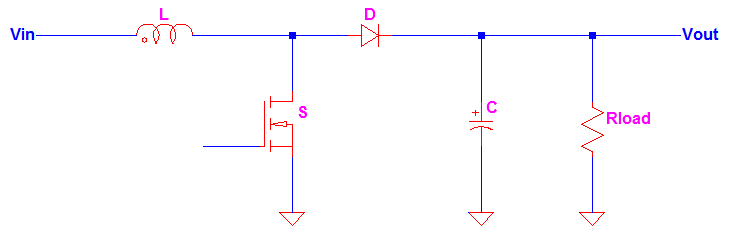## How to Calculate the Duty Cycle of Boost Converter

The inductor waveform is the key on how to calculate the duty cycle of boost converter. You can get direct equation for boost converter duty cycle formula from different sites but here I will discuss how it is derived. Meanwhile, a familiar boost converter schematic is shown in Figure 1. Figure 1 The inductor of the boost converter charges during